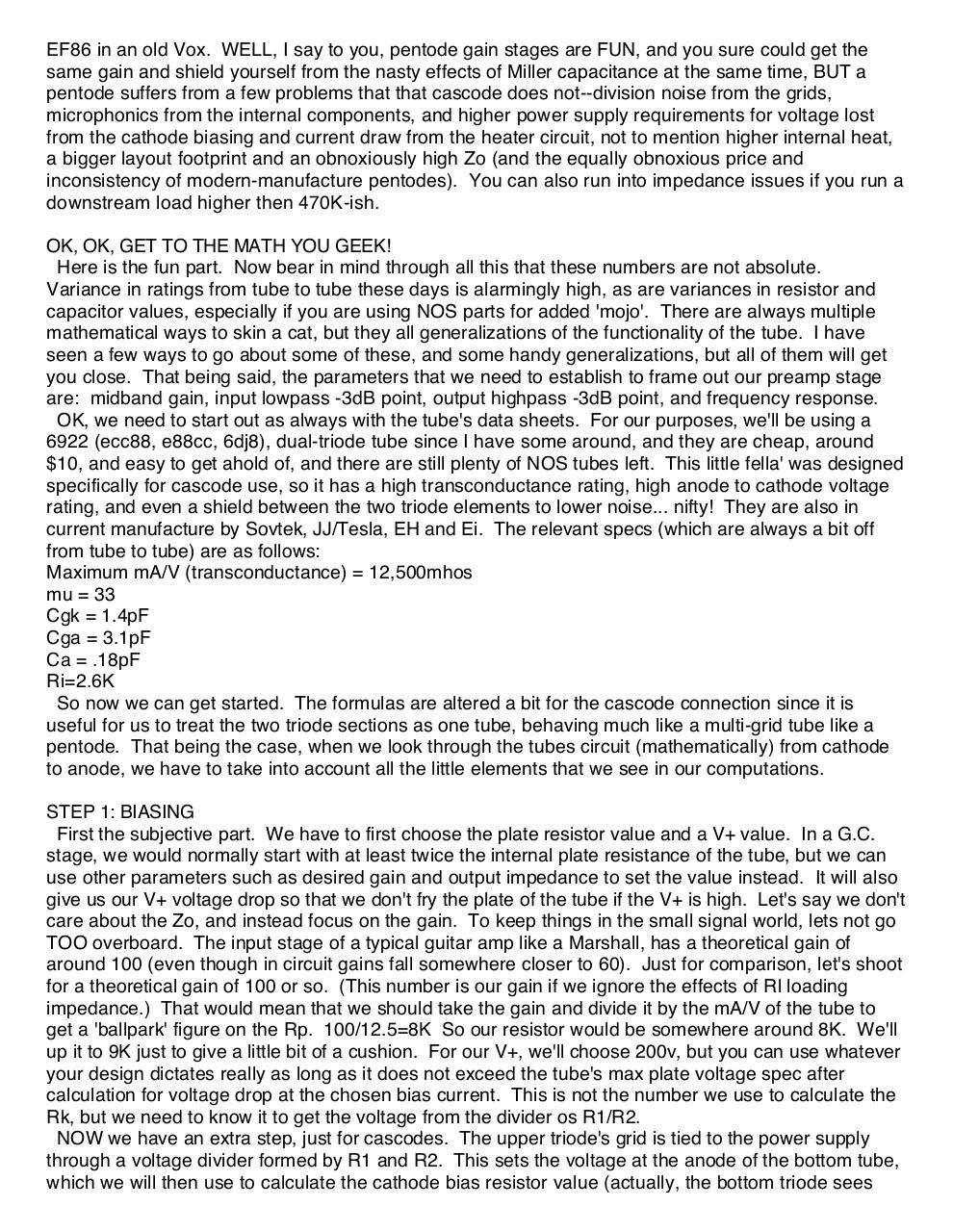# alex kenis cascodes.pdfPage 1 2 3 4 5 6

#### Text preview

EF86 in an old Vox. WELL, I say to you, pentode gain stages are FUN, and you sure could get the
same gain and shield yourself from the nasty effects of Miller capacitance at the same time, BUT a
pentode suffers from a few problems that that cascode does not--division noise from the grids,
microphonics from the internal components, and higher power supply requirements for voltage lost
from the cathode biasing and current draw from the heater circuit, not to mention higher internal heat,
a bigger layout footprint and an obnoxiously high Zo (and the equally obnoxious price and
inconsistency of modern-manufacture pentodes). You can also run into impedance issues if you run a
downstream load higher then 470K-ish.
OK, OK, GET TO THE MATH YOU GEEK!
Here is the fun part. Now bear in mind through all this that these numbers are not absolute.
Variance in ratings from tube to tube these days is alarmingly high, as are variances in resistor and
capacitor values, especially if you are using NOS parts for added 'mojo'. There are always multiple
mathematical ways to skin a cat, but they all generalizations of the functionality of the tube. I have
seen a few ways to go about some of these, and some handy generalizations, but all of them will get
you close. That being said, the parameters that we need to establish to frame out our preamp stage
are: midband gain, input lowpass -3dB point, output highpass -3dB point, and frequency response.
OK, we need to start out as always with the tube's data sheets. For our purposes, we'll be using a
6922 (ecc88, e88cc, 6dj8), dual-triode tube since I have some around, and they are cheap, around
\$10, and easy to get ahold of, and there are still plenty of NOS tubes left. This little fella' was designed
specifically for cascode use, so it has a high transconductance rating, high anode to cathode voltage
rating, and even a shield between the two triode elements to lower noise... nifty! They are also in
current manufacture by Sovtek, JJ/Tesla, EH and Ei. The relevant specs (which are always a bit off
from tube to tube) are as follows:
Maximum mA/V (transconductance) = 12,500mhos
mu = 33
Cgk = 1.4pF
Cga = 3.1pF
Ca = .18pF
Ri=2.6K
So now we can get started. The formulas are altered a bit for the cascode connection since it is
useful for us to treat the two triode sections as one tube, behaving much like a multi-grid tube like a
pentode. That being the case, when we look through the tubes circuit (mathematically) from cathode
to anode, we have to take into account all the little elements that we see in our computations.
STEP 1: BIASING
First the subjective part. We have to first choose the plate resistor value and a V+ value. In a G.C.
stage, we would normally start with at least twice the internal plate resistance of the tube, but we can
use other parameters such as desired gain and output impedance to set the value instead. It will also
give us our V+ voltage drop so that we don't fry the plate of the tube if the V+ is high. Let's say we don't
care about the Zo, and instead focus on the gain. To keep things in the small signal world, lets not go
TOO overboard. The input stage of a typical guitar amp like a Marshall, has a theoretical gain of
around 100 (even though in circuit gains fall somewhere closer to 60). Just for comparison, let's shoot
for a theoretical gain of 100 or so. (This number is our gain if we ignore the effects of Rl loading
impedance.) That would mean that we should take the gain and divide it by the mA/V of the tube to
get a 'ballpark' figure on the Rp. 100/12.5=8K So our resistor would be somewhere around 8K. We'll
up it to 9K just to give a little bit of a cushion. For our V+, we'll choose 200v, but you can use whatever
your design dictates really as long as it does not exceed the tube's max plate voltage spec after
calculation for voltage drop at the chosen bias current. This is not the number we use to calculate the
Rk, but we need to know it to get the voltage from the divider os R1/R2.
NOW we have an extra step, just for cascodes. The upper triode's grid is tied to the power supply
through a voltage divider formed by R1 and R2. This sets the voltage at the anode of the bottom tube,
which we will then use to calculate the cathode bias resistor value (actually, the bottom triode sees